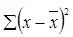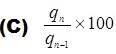Wednesday , September 29 2021
Breaking News

# Business Statistics Practice Test 1

Business Statistics Practice Test 1 designed Business Students specially for I.COM, BBA & MBA.

1) The word “Statistics” has been derived from English word:

(A)  Statistica

(B)  Status

(C)  Statistick

(D)  Static

Option C

2) A Numerical quantity computed from population is called:

(A)  Statistic

(B)  Attribute

(C)  Parameter

(D)  Body

Option C

3) A table has at least how many parts?

(A)  1

(B)  2

(C)  3

(D)  4

Option A

4) A pie chart consists of a ____subdivided into different sectors:

(A)  Circle

(B)  Triangle

(C)  Rectangle

(D)  Parabola

Option C

5) To find out size of shoes, the best average is:

(A)  G.M

(B)  H.M

(C)  Mode

(D)  None of these

Option B

6) If mean is 20, thenis:-

(A)  10

(B)  15

(C)  5

(D)  20

Option C

7) Index number for base period is always:

(A)  Zero

(B)  80

(C)  100

(D)  None

Option C

8) Link relatives are obtained by:

(A)(B)Option C

9) Pr (Diamond card) =

(A)  13/52

(B)  1/52

(C)  26/52

(D)  0

Option C

10)  (10) Probability is measurement of ______ :

(A)  Certainty

(B)  Uncertainty

(C)  Quality

(D)  None

Option C

11)   Now a day the World Statistic can be expressed in how many ways:

(A)       2

(B)       3

(C)       4

(D)       5

12)  Number of weeks in a month is an example of:

(A)       Discrete variable

(B)       Continuous variable

(C)       Attribute

(D)       Constant

13)   The process of arranging data into rows and columns is called:

(A)       Frequency distribution

(B)      Tabulation

(C)       Classification

(D)       Ogive

14)        A statistical table has at least:

(A)       One part

(B)       Two parts

(C)       Three parts

(D)       Four parts

15)        We must arrange the data before calculating:

(A)       A.M

(B)       Median

(C)       Mode

(D)       Mode

16)        Arithmetic Mean is based on:

(A)       Extreme values

(B)       Middle value

(C)       All the values

(D)       Most common value

17)        For averaging the relatives, we never use:

(A)       A.M

(B)       G.M

(C)       Median

(D)       Mode

18)        Fisher Index Number is of Laspeyre’s and Paasche’s Index Numbers:

(A)       A.M

(B)       G.M

(C)       Median

(D)       Mode

19) The Maximum sum of dots when a die is tossed two time is :

(A)       1

(B)       2

(C)       36

(D)       12

1.2.